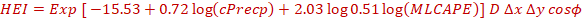## DESCRIPTION OF THUNDERSTORM INDICES BASED ON GEFS T1534 MODEL:

The Global Ensemble Forecast System with approximately 12.5km resolution (GEFS T1534) is run by IMD with 20 ensemble members and 1 control run. The model is run every day operationally with 00UTC initial conditions provided by NCMRWF and forecast of 10 days with 3hour interval is created. The flow chart of GEFS is shown in Figure 1.Figure 1. The Flow chart of Global Ensemble Forecast System (GEFS T1534 L64 ) developed at IITM and run by IMD.

By utilizing the GEFS model output , following probability indices are developed for thunderstorm prediction over Indian Region.

• ### 1. Hail Environmental Index (HEI):

Hail Environmental Index (Lepore et al 2018) is given by following equation:(1)

Where:
cPrecp is the convective precipitation
0-3km SRH is the Storm Relative Helicity (SRH) between 0-3km
MLCAPE is 180ha mixed layer Convective Available Potential Energy (CAPE)
D ∆x ∆y cosϕ is the offset which accounts for the varying grid area and month Length.
φ is the latitude in radians
∆x and ∆y are the longitude and latitude spacings in degrees respectively which are both equal to 0.125 0 respectively
The offset term makes the units of indices as number of events per unit area per day

### 2. SuperCell Composite Parameter (SCP):

It is defined by Carbin et al 2016 as:(2)

### 3. Layer Relative Humidity (LAYRH):

Layer Relative Humidity is defined by Rajeevan et al 2012 as layer mean relative humidity from 1000hPa to 100hPa

### 4. Surface Wind Gust :

Surface wind gust is the output parameter available directly from GEFS model.

The threshold for each indices used for calculating the probability of occurrence among each ensemble member is ---